mscroggs.co.uk
mscroggs.co.uksubscribe

# Blog

2019-11-24
This year, the front page of mscroggs.co.uk will once again feature an Advent calendar, just like last year, the year before, the year before and the year before. Behind each door, there will be a puzzle with a three digit solution. The solution to each day's puzzle forms part of a logic puzzle:
It's nearly Christmas and something terrible has happened: while out on a test flight, Santa's sled was damaged and Santa, Rudolph and Blitzen fell to the ground over the Advent Isles. You need to find Santa and his reindeer before Christmas is ruined for everyone.
You have gathered one inhabitant of the four largest Advent Isles—Rum, Land, Moon and County—and they are going to give you a series of clues about where Santa and his reindeer landed. However, one or more of the islanders you have gathered may have been involved in damaging Santa's sled and causing it to crash: any islander involved in this will lie to you to attempt to stop you from finding Santa and his reindeer. Once you are ready to search for Santa, Rudolph and Blitzen, you can find the map by following this link.
Each of the clues will be about Santa's, Rudolph's or Blitzen's positions in Advent Standard Coordinates (ASC): ASC are given by six two-digit numbers with dots inbetween, for example 12.52.12.13.84.55. For this example coordinate, the islanders will refer to (the first) 12 as the first coordinate, 52 as the second coordinate, (the second) 12 as the third coordinate, 13 as the fourth coordinate, 84 as the fifth coordinate, and 55 as the sixth coordinate.
To find a point's ASC coordinates, split a map of the islands into a 9×9 grid, then number the rows and columns 1 to 9: the first two digits of ASC give the vertical then horizontal position of a square in this grid. The next two digits then give a smaller square when this square is then itself split into a 9×9 grid, and so on. An example is show below.The ASC coordinates of this pair of flowers are 12.52.12.13.84.55 (click to enlarge).
Behind each day (except Christmas Day), there is a puzzle with a three-digit answer. Each of these answers forms part of a fact that one of the islanders tells you. You must use these clues to find Santa and his two reindeer.
Ten randomly selected people who solve all the puzzles, find Santa and his reindeer, and fill in the entry form behind the door on the 25th will win prizes!
The winners will be randomly chosen from all those who submit the entry form before the end of 2019. Each day's puzzle (and the entry form on Christmas Day) will be available from 5:00am GMT. But as the winners will be selected randomly, there's no need to get up at 5am on Christmas Day to enter!
To win a prize, you must submit your entry before the end of 2019. Only one entry will be accepted per person. If you have any questions, ask them in the comments below or on Twitter.
So once December is here, get solving! Good luck and have a very merry Christmas!

### Similar posts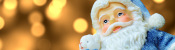Christmas (2018) is overChristmas (2018) is coming!Christmas (2017) is over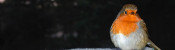Christmas (2017) is coming!

Comments in green were written by me. Comments in blue were not written by me.
This search space is so large, it's way harder than trying to find Wally like in the children's books. Best to read everything carefully looking for any clues, and then solve all the puzzles and logic before searching randomly!
Did you know Wally is called Waldo in the US, Walter in Germany, and Charlie in France?
Dr. Matrix
Thank you, I was worried. Thanks also for the puzzles, makes a change from my usual sudokus. I especially liked 3, 9, 11, 16, 21 and 23.
(anonymous)
@(anonymous): You don't get a confirmation email but if you hit submit you'll be entered. (I'll add confirmation emails before next year...)
Matthew
Do we get a confirmation email after submission of the entry form? I never received one, so I'm not sure if I am entered.
(anonymous)
@Seth: If you find them on the map then that's all you need to do. (And if you didn't find them yet then the entry form won't appear so you definably did everything you need to do.)
Matthew

Allowed HTML tags: <br> <a> <small> <b> <i> <s> <sup> <sub> <u> <spoiler> <ul> <ol> <li>
To prove you are not a spam bot, please type "width" in the box below (case sensitive):
2019-09-15
Today, I wrote this LaTeΧ document. It only uses default LaTeΧ functionality, and uses no packages.
If you compile it (twice so that cross references are not ??s), you will get a four page document (pages numbered i to iv), that repeatedly says: "This document ends on page v."This document ends on page iv and says: "This document ends on page v."
If you then recompile it, you will get a five page document (pages numbered i to v), that repeatedly says: "This document ends on page iv."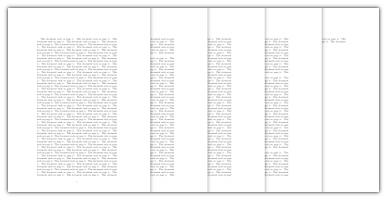This document ends on page v and says: "This document ends on page iv."
Repeatedly compiling it will alternately give these two results, and so the process of compiling this LaTeX document does not converge.
Tags: latex

### Similar posts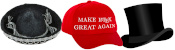realhats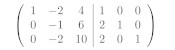Gaussian elimination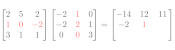Matrix multiplication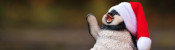Christmas (2019) is over

Comments in green were written by me. Comments in blue were not written by me.

Allowed HTML tags: <br> <a> <small> <b> <i> <s> <sup> <sub> <u> <spoiler> <ul> <ol> <li>
To prove you are not a spam bot, please type "p" then "r" then "i" then "m" then "e" in the box below (case sensitive):
2019-09-01
This week, I've been in Cambridge for Talking Maths in Public (TMiP). TMiP is a conference for anyone involved in—or interested in getting involved in—any sort of maths outreach, enrichment, or public engagement activity. It was really good, and I highly recommend coming to TMiP 2021.
The Saturday morning at TMiP was filled with a choice of activities, including a treasure punt (a treasure hunt on a punt) written by me. This post contains the puzzle from the treasure punt for anyone who was there and would like to revisit it, or anyone who wasn't there and would like to give it a try. In case you're not current in Cambridge on a punt, the clues that you were meant to spot during the punt are given behing spoiler tags (hover/click to reveal).

### Instructions

Each boat was given a copy of the instructions, and a box that was locked using a combination lock.
If you want to make your own treasure punt or similar activity, you can find the LaTeX code used to create the instructions and the Python code I used to check that the puzzle has a unique solution on GitHub. It's licensed with a CC BY 4.0 licence, so you can resuse an edit it in any way you like, as long as you attribute the bits I made that you keep.

### The puzzle

Four mathematicians—Ben, Katie, Kevin, and Sam—each have one of the four clues needed to unlock a great treasure. On a sunny/cloudy/rainy/snowy (delete as appropriate) day, they meet up in Cambridge to go punting, share their clues, work out the code for the lock, and share out the treasure. One or more of the mathematicians, however, has decided to lie about their clue so they can steal all the treasure for themselves. At least one mathematician is telling the truth. (If the mathematicians say multiple sentences about their clue, then they are either all true or all false.)
They meet at Cambridge Chauffeur Punts, and head North under Silver Street Bridge. Ben points out a plaque on the bridge with two years written on it:
"My clue," he says, "tells me that the sum of the digits of the code is equal to the sum of the digits of the earlier year on that plaque (the year is 1702). My clue also tells me that at least one of the digits of the code is 7."
The mathematicians next punt under the Mathematical Bridge, gasping in awe at its tangential trusses, then punt along the river under King's College Bridge and past King's College. Katie points to a sign on the King's College lawn near the river:
"See that sign whose initials are PNM?" says Katie. "My clue states that first digit of the code is equal to the number of vowels on that sign (The sign says "Private: No Mooring"). My clue also tells me that at least one of the digits of the code is 1."
They then reach Clare Bridge. Kevin points out the spheres on Clare Bridge:
"My clue," he says, "states that the total number of spheres on both sides of this bridge is a factor of the code (there are 14 spheres). My clue also tells me that at least one of the digits of the code is 2." (Kevin has not noticed that one of the spheres had a wedge missing, so counts that as a whole sphere.)
They continue past Clare College. Just before they reach Garret Hostel Bridge, Sam points out the Jerwood Library and a sign showing the year it was built (it was built in 1998):
"My clue," she says, "says that the largest prime factor of that year appears in the code (in the same way that you might say the number 18 appears in 1018 or 2189). My clue also says that the smallest prime factor of that year appears in the code. My clue also told me that at least one of the digits of the code is 0."
They then punt under Garret Hostel Bridge, turn around between it and Trinity College Bridge, and head back towards Cambridge Chauffeur Punts. Zut alors, the lies confuse them and they can't unlock the treasure. Can you work out who is lying and claim the treasure for yourself?

### The solution

The solution to the treasure punt is given below. Once you're ready to see it, click "Show solution".

### Similar postsChristmas card 2019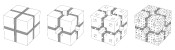Christmas card 2018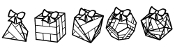Christmas card 2017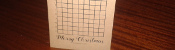Christmas card 2016

Comments in green were written by me. Comments in blue were not written by me.

Allowed HTML tags: <br> <a> <small> <b> <i> <s> <sup> <sub> <u> <spoiler> <ul> <ol> <li>
To prove you are not a spam bot, please type "pmuj" backwards in the box below (case sensitive):
2019-07-03
This year's Big Internet Math-Off is now underway with 15 completely new contestants (plus one returning contender). As I'm not the returning contestant, I haven't been spending my time preparing my pitches. Instead, I've spent my time making an unofficial Big Internet Math-Off sticker book.
To complete the sticker book, you will need to collect 162 different stickers. Every day, you will be given a pack of 5 stickers; there are also some bonus packs available if you can find them (Hint: keep reading).

### How many stickers will I need?

Using the same method as I did for last year's World Cup sticker book, you can work out that the expected number of stickers needed to finish the sticker book:
If you have already stuck $$n$$ stickers into your album, then the probability that the next sticker you get is new is
$$\frac{162-n}{162}.$$
The probability that the second sticker you get is the next new sticker is
$$\mathbb{P}(\text{next sticker is not new})\times\mathbb{P}(\text{sticker after next is new})$$ $$=\frac{n}{162}\times\frac{162-n}{162}.$$
Following the same method, we can see that the probability that the $$i$$th sticker you buy is the next new sticker is
$$\left(\frac{n}{162}\right)^{i-1}\times\frac{162-n}{162}.$$
Using this, we can calculate the expected number of stickers you will need to buy until you find a new one:
$$\sum_{i=1}^{\infty}i \left(\frac{162-n}{162}\right) \left(\frac{n}{162}\right)^{i-1} = \frac{162}{162-n}$$
Therefore, to get all 162 stickers, you should expect to buy
$$\sum_{n=0}^{161}\frac{162}{162-n} = 918 \text{ stickers}.$$
Using just your daily packs, it will take you until the end of the year to collect this many stickers. Of course, you'll only need to collect this many if you don't swap your duplicate stickers.

### How many stickers will I need if I swap?

To work out the expected number of stickers stickers you'd need if you swap, let's first think about two people who want to complete their stickerbooks together. If there are $$a$$ stickers that both collectors need and $$b$$ stickers that one collector has and the other one needs, then let $$E_{a,b}$$ be the expected number of stickers they need to finish their sticker books. The next sticker they get could be one of three things:
• A sticker they both need (with probability $$\frac{a}{162}$$);
• A sticker one of them needs (with probability $$\frac{b}{162}$$);
• A sticker they both have (with probability $$\frac{162-a-b}{162}$$).
Therefore, the expected number of stickers they need to complete their sticker books is
$$E_{a,b}=1+\frac{a}{162}E_{a-1,b+1}+\frac{b}{162}E_{a,b-1}+\frac{162-a-b}{162}E_{a,b}.$$
This can be rearranged to give
$$E_{a,b}= \frac{162}{a+b}+ \frac{a}{a+b}E_{a-1,b+1} +\frac{b}{a+b}E_{a,b-1}$$
We know that $E_{0,0}=0$ (as if $$a=0$$ and $$b=0$$, both collectors have already finished their sticker books). Using this and the formula above, we can work out that
$$E_{0,1}=162+E_{0,0}=162$$ $$E_{1,0}=162+E_{0,1}=324$$ $$E_{0,2}=\frac{162}2+E_{0,1}=243$$ $$E_{1,1}=\frac{162}2+\frac12E_{0,2}+\frac12E_{1,0}=364.5$$
... and so on until we find that $$E_{162,0}=1269$$, and so our collectors should expect to collect 634 stickers each to complete their sticker books.
For three people, we can work out that if there are $$a$$ stickers that all three need, $$b$$ stickers that two need, and $$c$$ stickers that one needs, then
$$E_{a,b,c} = \frac{162}{a+b+c}+ \frac{a}{a+b+c}E_{a-1,b+1,c} +\frac{b}{a+b+c}E_{a,b-1,c+1} +\frac{c}{a+b+c}E_{a,b,c-1}.$$
In the same way as for two people, we find that $$E_{162,0,0}=1572$$, and so our collectors should expect to collect 524 stickers each to complete their sticker books.
Doing the same thing for four people gives an expected 463 stickers required each.
After four people, however, the Python code I wrote to do these calculations takes too long to run, so instead I approximated the numbers by simulating 500 groups of $$n$$ people collecting stickers, and taking the average number of stickers they needed. The results are shown in the graph below.
The red dots are the expected values we calculated exactly, and the blue crosses are the simulated values. It looks like you'll need to collect at least 250 stickers to finish the album: in order to get this many before the end of the Math-Off, you'll need to find 20 bonus packs...
Of course, these are just the mean values and you could get lucky and need fewer stickers. The next graph shows box plots with the quartiles of the data from the simulations.
So if you're lucky, you could complete the album with fewer stickers or fewer friends.
As a thank you for reading to the end of this blog post, here's a link that will give you two bonus packs and help you on your way to the 250 expected stickers...

### Similar posts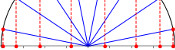Runge's PhenomenonWorld Cup stickers 2018, pt. 3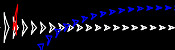MathsteroidsWorld Cup stickers 2018, pt. 2

Comments in green were written by me. Comments in blue were not written by me.
Link to sticker book, in the first paragraph, does not work. It points to mathoffstickbook.com
Pat Ashforth
minor typo for the 2 collector case

> and so our collectors should expect to collect 364 stickers

should be 634.

Allowed HTML tags: <br> <a> <small> <b> <i> <s> <sup> <sub> <u> <spoiler> <ul> <ol> <li>
To prove you are not a spam bot, please type "hparg" backwards in the box below (case sensitive):
2019-06-19
Last night at MathsJam, Peter Kagey showed me a conjecture about OEIS sequence A308092.A308092
The sum of the first $$n$$ terms of the sequence is the concatenation of the first $$n$$ bits of the sequence read as binary, with $$a(1) = 1$$.
1, 2, 3, 7, 14, 28, 56, 112, 224, 448, 896, 1791, 3583, 7166, ...To understand this definition, let's look at the first few terms of this sequence written in binary:
1, 10, 11, 111, 1110, 11100, 111000, 1110000, 11100000, 111000000, ...
By "the concatenation of the first $$n$$ bits of the sequence", it means the first $$n$$ binary digits of the whole sequence written in order: 1, then 11, then 110, then 1101, then 11011, then 110111, and so on. So the definition means:
• The first term is 1, as given in the definition ($$a(1)=1$$).
• The sum of the first 2 terms is the first 2 digits: $$1+10=11$$.
• The sum of the first 3 terms is the first 3 digits: $$1+10+11=110$$.
• The sum of the first 4 terms is the first 4 digits: $$1+10+11+111=1101$$.
• The sum of the first 5 terms is the first 5 digits: $$1+10+11+111+1110=11011$$.
As we know that the sum of the first $$n-1$$ terms is the first $$n-1$$ digits, we can calculate the third term of this sequence onwards using: "$$a(n)$$ is the concatenation of the first $$n$$ bits of the sequence subtract concatenation of the first $$n-1$$ bits of the sequence":
• The third term is $$110 - 11 = 11$$.
• The fourth term is $$1101 - 110 = 111$$.
• The fourth term is $$11011 - 1101 = 1110$$.
• The fifth term is $$110111 - 11011 = 11100$$.

### The conjecture

Peter's conjecture is that the number of 1s in each term is greater than or equal to the number of 1s in the previous term.
I'm going to prove this conjecture. If you'd like to have a try first, stop reading now and come back when you're ready for spoilers. (If you'd like a hint, read the next section then pause again.)

The third term of the sequence onwards can be calculated by subtracting the first $$n-1$$ digits from the first $$n$$ digits. If the first $$n-1$$ digits form a binary number $$x$$, then the first $$n$$ digits will be $$2x+d$$, where $$d$$ is the $$n$$th digit (because moving all the digits to the left one place in binary is multiplying by two).
Therefore the different is $$2x+d-x=x+d$$, and so we can work out the $$n$$th term of the sequence by adding the $$n$$th digit in the sequence to the first $$n-1$$ digits. (Hat tip to Martin Harris, who spotted this first.)

### Carrying

Adding 1 to a binary number the ends in 1 will cause 1 to carry over to the left. This carrying will continue until the 1 is carried into a position containing 0, and after this all the digits to the left of this 0 will remain unchanged.
Therefore adding a digit to the first $$n-1$$ digits can only change the digits from the rightmost 0 onwards.

### Endings

We can therefore disregard all the digits before the rightmost 0, and look at how the $$n$$th term compares to the $$(n-1)$$th term. There are 5 ways in which the first $$n$$ digits could end:
• $$00$$
• $$010$$
• $$01...10$$ (where $$1...1$$ is a string of 2 or more ones)
• $$01$$
• $$01...1$$ (where $$1...1$$ is again a string of 2 or more ones)
We now look at each of these in turn and show that the $$n$$th term will contain at least as many ones at the $$(n-1)$$th term.

#### Case 1: $$00$$

If the first $$n$$ digits of the sequence are $$x00$$ (a binary number $$x$$ followed by two zeros), then the $$(n-1)$$th term of the sequence is $$x+0=x$$, and the $$n$$th term of the sequence is $$x0+0=x0$$. Both $$x$$ and $$x0$$ contain the same number of ones.

#### Case 2: $$010$$

If the first $$n$$ digits of the sequence are $$x010$$, then the $$(n-1)$$th term of the sequence is $$x0+1=x1$$, and the $$n$$th term of the sequence is $$x01+0=x01$$. Both $$x1$$ and $$x01$$ contain the same number of ones.

#### Case 3: $$01...10$$

If the first $$n$$ digits of the sequence are $$x01...10$$, then the $$(n-1)$$th term of the sequence is $$x01...1+1=x10...0$$, and the $$n$$th term of the sequence is $$x01...10+1=x01...1$$. $$x01...1$$ contains more ones than $$x10...0$$.

#### Case 4: $$01$$

If the first $$n$$ digits of the sequence are $$x01$$, then the $$(n-1)$$th term of the sequence is $$x+0=x$$, and the $$n$$th term of the sequence is $$x0+1=x1$$. $$x1$$ contains one more one than $$x$$.

#### Case 5: $$01...1$$

If the first $$n$$ digits of the sequence are $$x01...1$$, then the $$(n-1)$$th term of the sequence is $$x01...1+1=x10...0$$, and the $$n$$th term of the sequence is $$x01...1+1=x10...0$$. Both these contain the same number of ones.

In all five cases, the $$n$$th term contains more ones or an equal number of ones to the $$(n-1)$$th term, and so the conjecture is true.

### Similar postsMathsteroidsBuilding MENACEs for other gamesBig Ben Strikes Again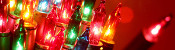Christmas (2016) is over

Comments in green were written by me. Comments in blue were not written by me.